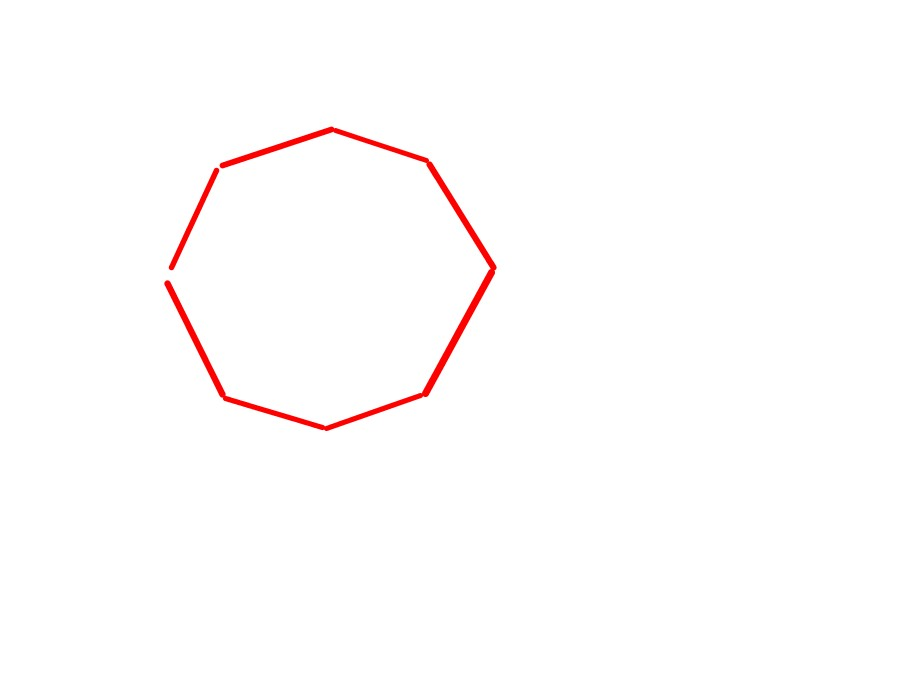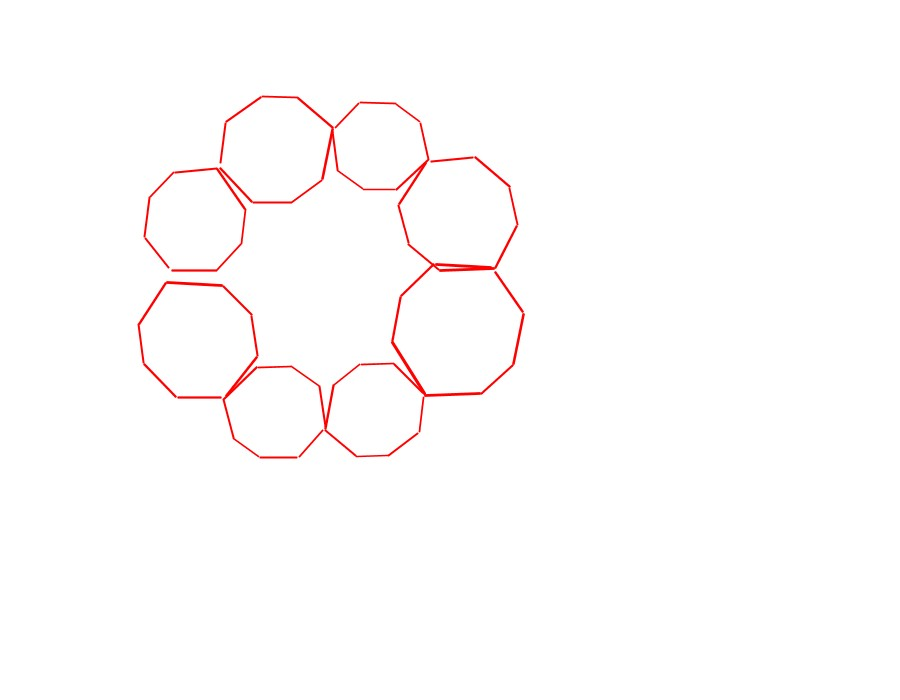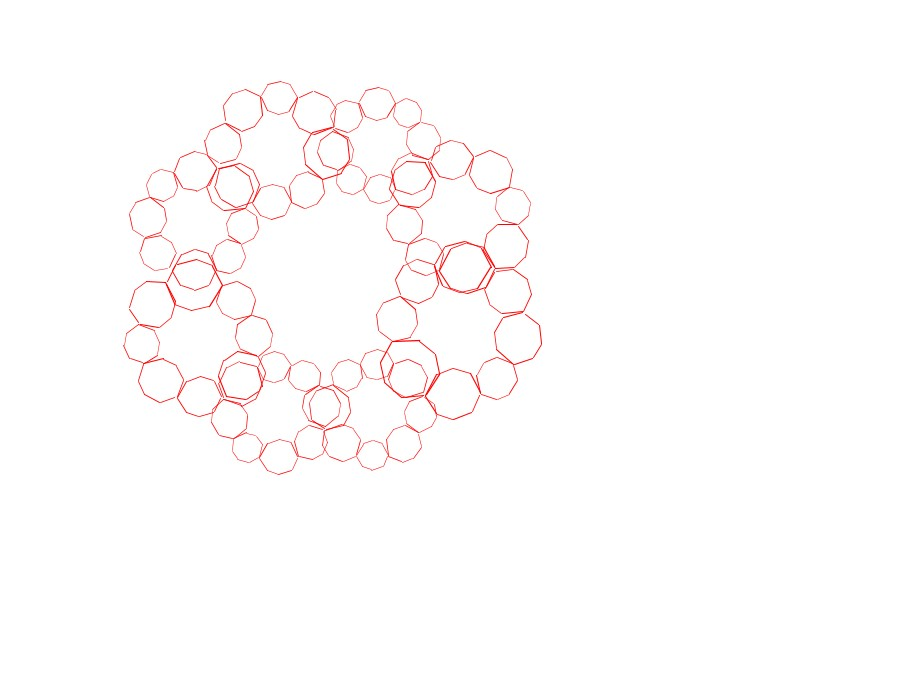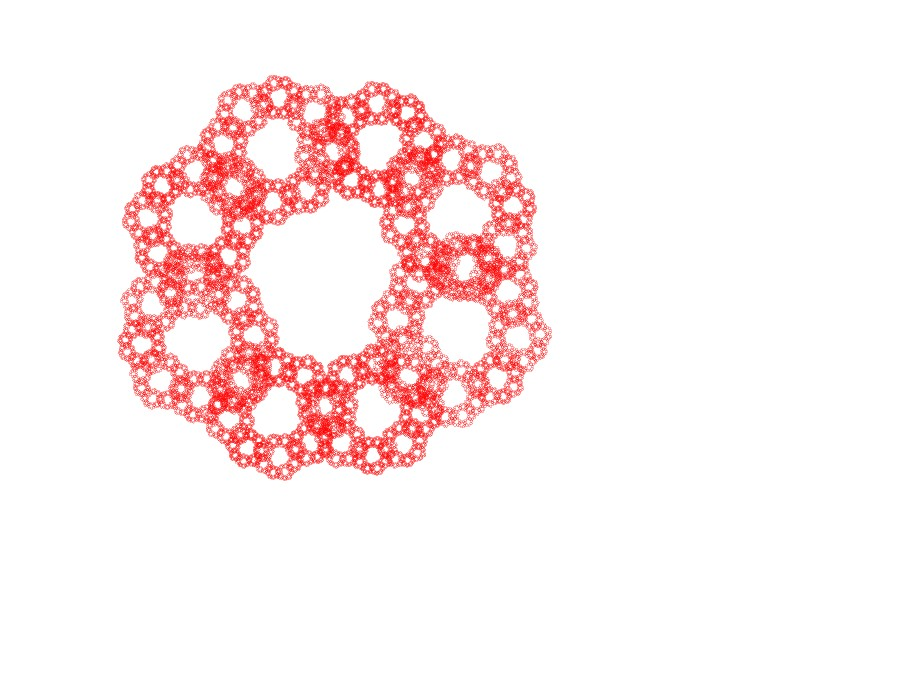## 1. A fern is like a fern

Fractals are based on the notion of self-similarity. Self-similarity is when things are like themselves. One example is a fern. The branch of a fern looks like a whole fern. The leaf of a fern looks like the branch of a fern, which looks like a whole fern.## 2. A mathematical fern

The self-similarity of a real fern is limited. But the fact that a fern has self-similar characteristics means that we can create a fern-like object with a few mathematical rules, such as the Barnsley fern.## 3. A simple fractalReplace each of the lines with the entire drawing, at the scale of that line:Keep going...And eventually you will have an object which is self-similar at many scales. These objects tend to have a certain naturalness or harmony about them.## 4. Fractals in time

If we use a fractal that's in one dimension, such as the Cantor Set, then we can read off the points of the fractal as rhythmic elements, as if it were a score over time. By varying the ratios of this fractal, we can create more interesting rhythms. This is the core of Fractal Music Machine's algorithm.Cantor Set: try reading off the bottom line as a rhythm.

## 5. Fractal Music Machine in action

The video below shows Fractal Music Machine creating a fractal. It takes pattern of notes (Recursive depth 1) and, for each note, repeats the whole pattern (recursive depth 1->2), and does this repeatedly a few times to get a much deeper pattern. The pattern of notes includes frequencies, which are multiplied by each other to obtain new frequencies as the pattern evolves.

## 6. Fractal music elsewhere on the web

Here is a small selection of some other interesting links to fractal music experiments and research: